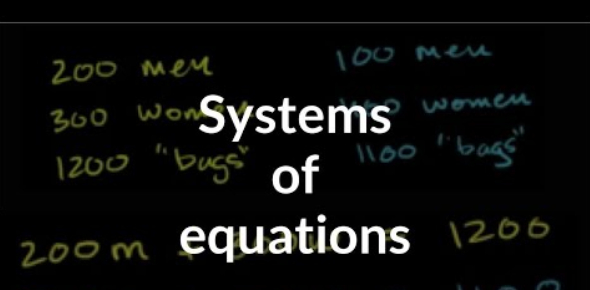# Math Quiz: Systems Of Equations!

8 Questions | Attempts: 96SettingsAre you ready for a maths quiz about the systems of equations? A system of equation is formed when one has two equations with the same set of the unknown, and a student is expected to find the unknown. One can use three ways to solve linear equations in two variables graphing, substitution method, or elimination method. Do take up the quiz and get to see how well you will use these methods to solve the equations below.

• 1.
What is the first step you should do when solving by elimination?
• A.

• B.

Multiply each equation by a constant

• C.

Make sure the variables are aligned

• D.

Distribute

• 2.
Graph the system of equations to determine whether it has no solution, many solutions, or one solution. If the system has one solution, name it.  3x - 2y = -1 2x + y = 4
• A.

One solution at (2, 1)

• B.

One solution at (1, 2)

• C.

No solution

• D.

Infinitely many solutions

• 3.
• A.

No solution, multiplication

• B.

• C.

Many solutions, substitution

• D.

• 4.
Solve by substitution of the following system. 2x + 3y = 3 x - y = 9
• A.

(30,21)

• B.

(21,30)

• C.

(6, -3)

• D.

(-24, -15)

• 5.
2x2+ 4x + 2=0
• A.

-5

• B.

-1

• C.

5

• D.

40

• E.

9

• 6.
How many possible types of solutions do you have when you solve a system of equations?
• A.

1

• B.

2

• C.

3

• D.

4

• 7.
A line has a gradient -2/3 and passes through the point (3,0). Find the equation of the line.
• A.

Y = - 2/3 x + 3

• B.

Y = - 2/3 x + 2

• C.

Y = 2/3 x + 2

• D.

Y = 2/3 x + 3

• 8.
( - 4, 4) and ( 0, 3) are points on the line l. State the equation of the line l.
• A.

Y=1.75x

• B.

Y=1.75x + 0.75

• C.

Y=4/7x + 3

• D.

None of the above

## Related TopicsBack to top
×

Wait!
Here's an interesting quiz for you.